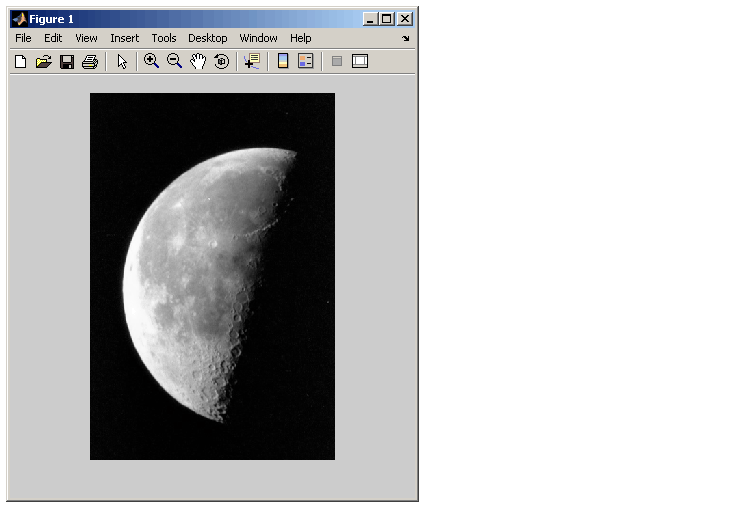Image Processing Toolbox User's GuideUsing imshow to Display Images

You can use the `imshow` function to display an image that has already been imported into the MATLAB workspace or to display an image stored in a graphics file. For example, this code reads an image into the MATLAB workspace and then displays it in a MATLAB figure window.

• ```moon = imread('moon.tif');
imshow(moon);
```

The `imshow` function displays the image in a MATLAB figure window, as shown in the following figure.Image Displayed in a Figure Window by imshow

The `imshow` filename syntax

• ```imshow('moon.tif');
```

can be useful for scanning through images. Note, however, that when you use this syntax, the image data is not stored in the MATLAB workspace. If you want to bring the image into the workspace, you must use the `getimage` function, which retrieves the image data from the current Handle Graphics image object. For example,

• ``````moon = getimage;
``````

assigns the image data from `moon.tif `to the variable `moon` if the figure window in which it is displayed is currently active.

For more information about using `imshow`, see these additional topics.

For more information about using `imshow` to display the various image types supported by the toolbox, see Displaying Different Image Types.Understanding Handle Graphics Object Property Settings Specifying the Initial Image Magnification